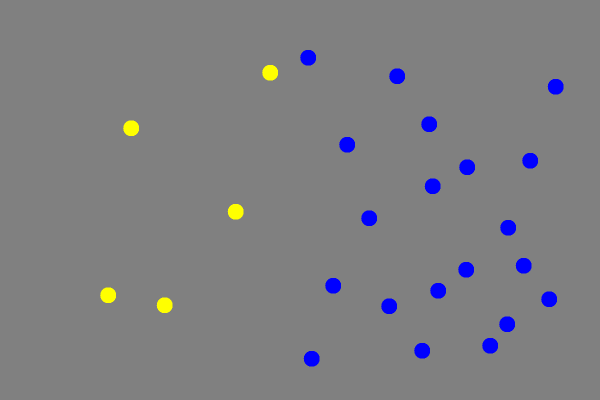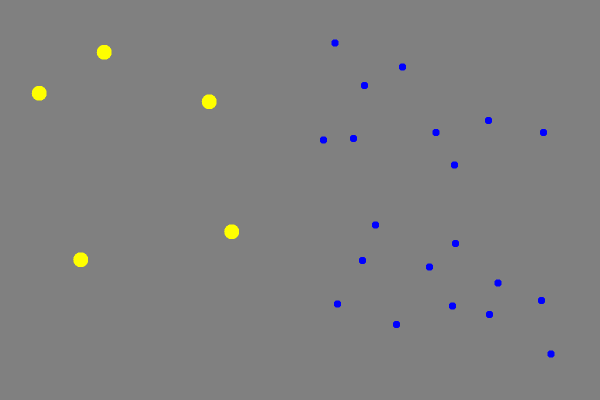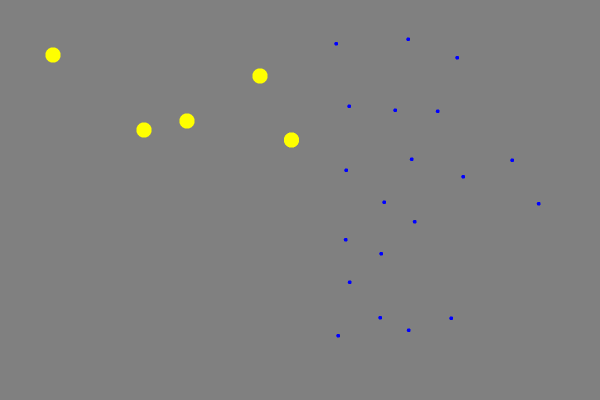# Panamath Software Manual

(Difference between revisions)
 Revision as of 18:36, 6 October 2011 (view source)Sharon (Talk | contribs) (→General Settings)← Older edit Revision as of 18:40, 6 October 2011 (view source)Sharon (Talk | contribs) (→Display)Newer edit → Line 78: Line 78: ==Display== ==Display== + *Between trials show: + **There are three options {Fixation cross, Number of trials remaining, Nothing} + **Fixation cross displays a cross in the center of the screen between trials. + **Number of trials remaining displays the number of remaining trials in the test between trials. + **Nothing displays a blank screen between trials. + *Show Dot Window Rectangles: + **If this option is selected, outlines of the window in which the dot sets will appear will be displayed on the screen during the test. + *Show instructions text during test ==Kids Characters== ==Kids Characters==

# Subject ID

-talk about the point of subject id here

# Age

-talk about age. importance. does it matter if you put the wrong age. consequences of doing so. etc.

# How Ratio Bins Are Calculated From Age And Difficulty

If the user has not entered customized settings under Advanced Options on the main panel then the program will generate appropriate settings for numerosity ratio and display time. It does this by taking the users age and selected difficulty, adjusting the age based on the difficulty, then finding appropriate values for numerosity ratio and display time using this adjusted age. The following sections show exactly how adjusted age, numerosity ratio, and display time are calculated.

• Very Easy Difficulty
• If user's age is between 0 and 20, then the formula .5 * age is used. If the user is between 20 and 40, then 3/20 * age + 7 is used. Otherwise, an adjusted age of 13 is used. In all subsequent algorithms, this adjusted age is used instead of the real age. This casuses the numerosity ratio and display time to scale with age and difficulty.
• Easy Difficulty
• If user's age is between 0 and 20, then the formula 3/5 * age is used. If the user is between 20 and 40, then 3/20 * age + 9 is used. Otherwise, an adjusted age of 15 is used.
• Medium Difficulty
• On medium difficulty, the user's real age is used in all calculations. This means that a 20 year old will have an adjusted age of 20, a 40 year old will have an adjusted age of 40, etc.
• Hard Difficulty
• On hard difficulty, the adjusted age is given by the formula 4.116713430316e-01 + 1.111762903248e+00 * actualAge + 9.684975424473e-03 * actualAge^2. Note that at this difficulty, this formula is always used, unlike for very easy and easy difficulty, in which after 40 the same value is used.
• Very Hard Difficulty
• On very had difficulty, the adjusted age is given by the formula 4.755221349509e-01 + 1.477883254935e+00 * actualAge+ 1.382024390740e-02 * actualAge^2. This formula is used for all input ages.

## Numerosity Ratio

Once the adjusted age has been calculated by the above formulas, it is run through a function to determine appropriate numerosity ratios based on the adjusted age and the difficulty. When calculating the numerosity ratios, the weber fraction is used. The [Fraction] is the amount of change in a stimulus relative to the background "noise" in the stimulus. The important thing about the weber fraction is that it is roughly constant for a certain stimulus. In vision lab experiments, the weber fraction for panamath experiments is approximately weberFraction = adjustedAge ^ -.55. This value is used to calculate scaled values for numerosity ratio according to the following rules:

• If the adjusted age is less than 40 or is on very hard or hard difficulty, then the following four values are used as the numerosity ratios.
• (.75*w) + 1
• (1.25*w) + 1
• (2*w) + 1
• 2*(w + 1)
• If the adjusted age is greater than 40 and the user is on very easy, easy, or medium difficulty, then the following values are used for numerosity ratio, based on difficulty.
• Very Easy Difficulty
• 1.5
• 1.8
• 2.8
• 4.0
• Easy Difficulty
• 1.4
• 1.5
• 2.5
• 3.5
• Medium Difficulty
• 1.2
• 1.3
• 1.8
• 3.0

## Display Time

Display time is the simplest to calculate. It is based only on adjusted age, and is calculated according to the following rules:

• If the user's adjusted age is between 0 and 20, it is calculated according to the formula 3.23713 * e^(-0.0892973 * adjustedAge) + 0.0573327.
• If adjusted age is between 20 and 40, a display time of .6 seconds is used
• If adjusted age is between 40 and 70, display time is calculated according to the formula 0.0467 * adjustedAge - 1.2667
• If adjusted age is greater than 70, then display time is set to 2 seconds

Panamath can be fully customized using the Advanced Settings panel, which can be accessed by clicking "Set Advanced Options" at the bottom of the first screen that appears when Panamath is run.

The Advanced Settings panel is divided into six sections: General, Display, Kids Characters, Dot Colors, Response Keys, and Customize. Navigate between these sections by clicking on the section name on the left side of the panel (under the panamath logo). For each section, there is a "Restore Defaults" button. Clicking on this button resets all settings to defaults on that section only.

## General Settings

• Difficulty
• There are five levels of difficulty on Panamath {Very Easy, Easy, Medium, Hard, Very Hard}.
• Sound Feedback
• Choice for sound feedback are {Beep, Voice, None}.
• Sound feedback is the sound that is heard after each response during the test.
• The Beep setting and Voice setting both indicate to the participant whether or not the response was correct.
• Skip Instructions
• Selecting this check box skips the instructions screen that appears immediately after clicking "Start Experiment."
• Save Results
• Check this box to indicate whether or not the results of the test should be saved.
• If saving is selected, the Subject ID and the location of the results files (.xls and PDF) can be selected by editing the appropriate fields.

## Display

• Between trials show:
• There are three options {Fixation cross, Number of trials remaining, Nothing}
• Fixation cross displays a cross in the center of the screen between trials.
• Number of trials remaining displays the number of remaining trials in the test between trials.
• Nothing displays a blank screen between trials.
• Show Dot Window Rectangles:
• If this option is selected, outlines of the window in which the dot sets will appear will be displayed on the screen during the test.
• Show instructions text during test

## Kids Characters

In the kids characters section, there is the option to turn on/off the display of Sesame Street characters on the screen during the test. (By default, this setting is only on for participants who have entered an age of 7 or younger) This panel also allows selection of 2 of the 15 Sesame Street Characters to be associated with each dot set. To select a character for dot set 1, select the button next to the character on the left side. Similarly for dot set 2, select the character on the right side. Characters selected for dot set 1 and dots set 2 must be different. (By default, Big Bird is selected for dot set 1 and Grover is selected for dot set 2.)

## Dot Colors

In the dot colors section, the colors for the dot set 1, dot set 2, and the background can be changed by clicking on the colored rectangle, and then selecting the desired color.

## Response Keys

In the response keys section, the keys used to respond to either dot set, and also to advance to the next trial can be customized. To select a new key, click on the rectangle displaying the current key, and then press the new key that will be used.

## Customize

In the Advanced Settings Customize panel, the following parameters: dots window size and separation,size control, numerosity ratio, display time, number of dots, base dot size and average variation can be changed.

## How Size Controlling Works

Size control changes the average size of the dots in relation to the number of dots of each color.

Without size control, it would be possible to get most of the trials correct by simply noting which color fills up more area on the screen. A size control of 0, Non Size-Controlled, creates dots with the same average size for both sets of dots, while a negative size control creates smaller dots for the set with more dots, and a positive size control creates larger dots for the set with more dots.

A size control of -1,Size-Controlled, changes the average size of each dot set so that the area of the screen filled by each dot set is approximately equal.

If all the trials in a test used the same size control, it would be possible to get most of the trials correct by paying attention to either the average sizes of the dots or the area of the screen filled with each color. By using a combination of different size controls, we can ensure that the area and size of the dots will not reveal the correct answer. The size control number, which can be adjusted from -3 to 1 in the customize panel of the advanced settings panel, is the exponent to which the ratio of the number of dots is raised to obtain the ratio of the average sizes of dots.

### Non Size-Controlled

In a non size-controlled experiment, size control is 0. The ratio of the average sizes of the dots is calculated to be (numerosity ratio)0, which means that the ratio of the average sizes of the two dot sets is 1 for any numerosity ratio. This results in total area of each color being proportional to the number of dots.### Size-Controlled

In a size-controlled experiment, size control is -1. The ratio of the average sizes of the dots is (numerosity ratio)-1. With this setting, the total area that a one dot set fills will be the same as the other dot set, pixel ratio of the two colors is approximately 1:1.
In the example below, there are 5 yellow dots and 20 blue dots. The ratio of the sizes of dots is (1/4)-1 or (4/1). The yellow dots to blue dots average size ratio is 4:1.### Anti-Correlated

In an anti-correlated experiment, size control is -2. The ratio of the average sizes of the dots is (numerosity ratio)-2. With this setting, the total area that the dot set with less dots fills will be more than the area of the dot set with more dots.\\ In the example below, there are 5 yellow dots and 20 blue dots. The ratio of the sizes of dots is (1/4)-2 or (16/1). The yellow dots to blue dots average size ratio is 16:1.# Basics of the Program

-talk about basics of the actual program, including how to go between trials, how to respond, etc. very basic stuff.

## Questions

If you have any questions on how to use Panamath, please send us a message or e-mail us directly at panamath@panamath.org.